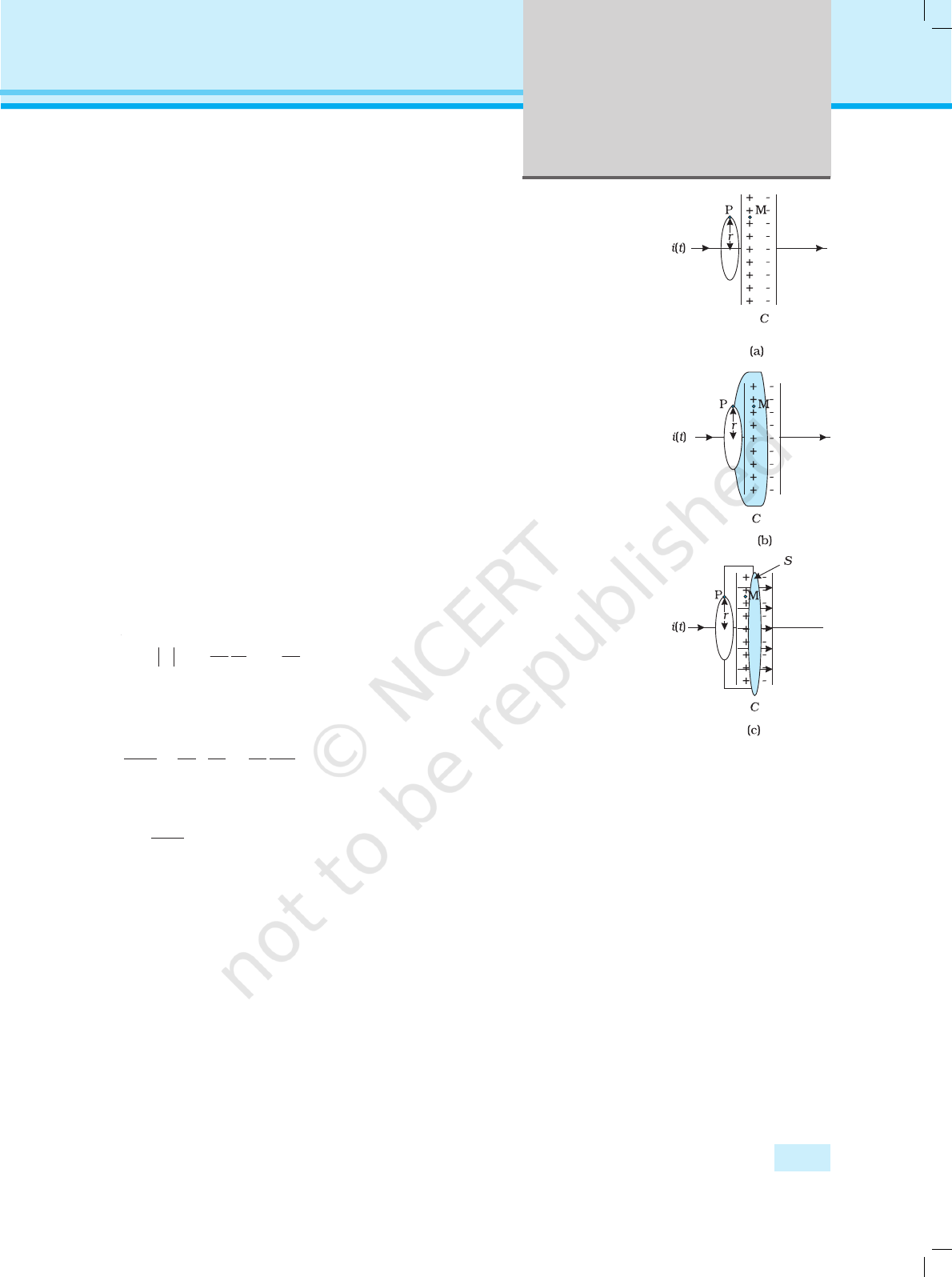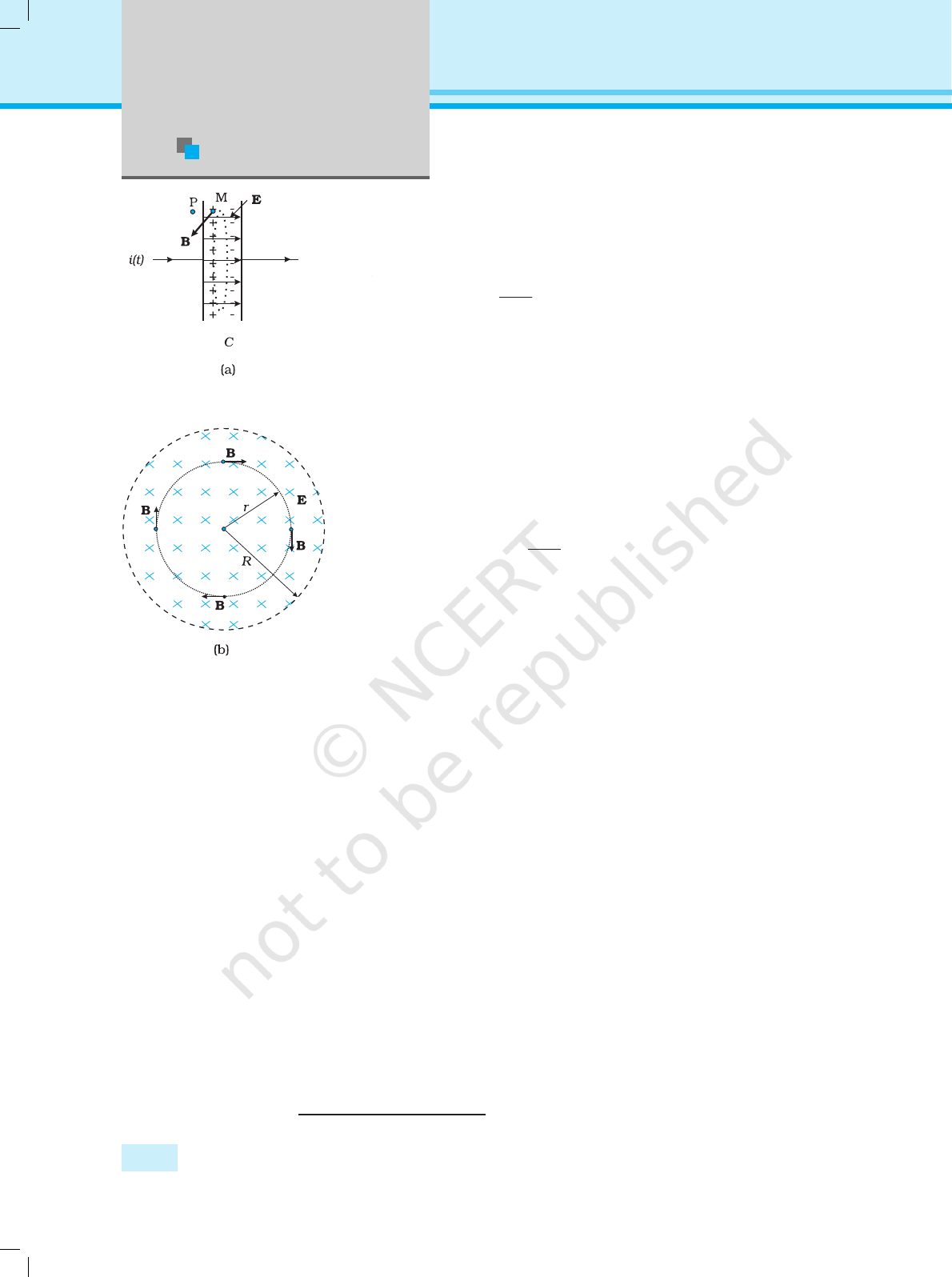Chapter Eight
ELECTROMAGNETIC
WAVES
8.1 INTRODUCTION
In Chapter 4, we learnt that an electric current produces magnetic field
and that two current-carrying wires exert a magnetic force on each other.
Further, in Chapter 6, we have seen that a magnetic field changing with
time gives rise to an electric field. Is the converse also true? Does an
electric field changing with time give rise to a magnetic field? James Clerk
Maxwell (1831-1879), argued that this was indeed the case – not only
an electric current but also a time-varying electric field generates magnetic
field. While applying the Ampere’s circuital law to find magnetic field at a
point outside a capacitor connected to a time-varying current, Maxwell
noticed an inconsistency in the Ampere’s circuital law. He suggested the
existence of an additional current, called by him, the displacement
current to remove this inconsistency.
Maxwell formulated a set of equations involving electric and magnetic
fields, and their sources, the charge and current densities. These
equations are known as Maxwell’s equations. Together with the Lorentz
force formula (Chapter 4), they mathematically express all the basic laws
of electromagnetism.
The most important prediction to emerge from Maxwell’s equations
is the existence of electromagnetic waves, which are (coupled) time-
varying electric and magnetic fields that propagate in space. The speed
of the waves, according to these equations, turned out to be very close to
2020-21Physics
270
the speed of light( 3 ×10
8
m/s), obtained from optical
measurements. This led to the remarkable conclusion
that light is an electromagnetic wave. Maxwell’s work
thus unified the domain of electricity, magnetism and
light. Hertz, in 1885, experimentally demonstrated the
existence of electromagnetic waves. Its technological use
by Marconi and others led in due course to the
revolution in communication that we are witnessing
today.
In this chapter, we first discuss the need for
displacement current and its consequences. Then we
present a descriptive account of electromagnetic waves.
The broad spectrum of electromagnetic waves,
stretching from γ rays (wavelength ~10
–12
m) to long
6
m) is described. How the
electromagnetic waves are sent and received for
communication is discussed in Chapter 15.
8.2 DISPLACEMENT CURRENT
We have seen in Chapter 4 that an electrical current
produces a magnetic field around it. Maxwell showed
that for logical consistency, a changing electric field must
also produce a magnetic field. This effect is of great
importance because it explains the existence of radio
waves, gamma rays and visible light, as well as all other
forms of electromagnetic waves.
To see how a changing electric field gives rise to
a magnetic field, let us consider the process of
charging of a capacitor and apply Ampere’s circuital
law given by (Chapter 4)
B
.
dl =
µ
0
i (t) (8.1)
to find magnetic field at a point outside the capacitor.
Figure 8.1(a) shows a parallel plate capacitor C which
is a part of circuit through which a time-dependent
current i (t) flows . Let us find the magnetic field at a
point such as P, in a region outside the parallel plate
capacitor. For this, we consider a plane circular loop of
radius r whose plane is perpendicular to the direction
of the current-carrying wire, and which is centred
symmetrically with respect to the wire [Fig. 8.1(a)]. From
symmetry, the magnetic field is directed along the
circumference of the circular loop and is the same in
magnitude at all points on the loop so that if B is the
magnitude of the field, the left side of Eq. (8.1) is B (2
π
r).
So we have
B (2πr) =
µ
0
i (t) (8 .2)
JAMES CLERK MAXWELL (1831–1879)
James Clerk Maxwell
(1831 – 1879) Born in
Edinburgh, Scotland,
was among the greatest
physicists of the
nineteenth century. He
derived the thermal
velocity distribution of
molecules in a gas and
was among the first to
obtain reliable
estimates of molecular
parameters from
measurable quantities
like viscosity, etc.
Maxwell’s greatest
acheivement was the
unification of the laws of
electricity and
magnetism (discovered
by Coulomb, Oersted,
into a consistent set of
equations now called
Maxwell’s equations.
From these he arrived at
the most important
conclusion that light is
an electromagnetic
wave. Interestingly,
Maxwell did not agree
with the idea (strongly
suggested by the
electrolysis) that
electricity was
particulate in nature.
2020-21271
Electromagnetic
Waves
Now, consider a different surface, which has the same boundary. This
is a pot like surface [Fig. 8.1(b)] which nowhere touches the current, but
has its bottom between the capacitor plates; its mouth is the circular
loop mentioned above. Another such surface is shaped like a tiffin box
(without the lid) [Fig. 8.1(c)]. On applying Ampere’s circuital law to such
surfaces with the same perimeter, we find that the left hand side of
Eq. (8.1) has not changed but the right hand side is zero and not
µ
0
i,
since no current passes through the surface of Fig. 8.1(b) and (c). So we
have a contradiction; calculated one way, there is a magnetic field at a
point P; calculated another way, the magnetic field at P is zero.
Since the contradiction arises from our use of Ampere’s circuital law,
this law must be missing something. The missing term must be such
that one gets the same magnetic field at point P, no matter what surface
is used.
We can actually guess the missing term by looking carefully at
Fig. 8.1(c). Is there anything passing through the surface S between the
plates of the capacitor? Yes, of course, the electric field! If the plates of the
capacitor have an area A, and a total charge Q, the magnitude of the
electric field E between the plates is (Q/A)/ε
0
(see Eq. 2.41). The field is
perpendicular to the surface S of Fig. 8.1(c). It has the same magnitude
over the area A of the capacitor plates, and vanishes outside it. So what
is the electric flux
Φ
E
through the surface S ? Using Gauss’s law, it is
E
0 0
1
= =
Q Q
A A
A
Φ
ε ε
=E
(8.3)
Now if the charge Q on the capacitor plates changes with time, there is a
current i = (dQ/dt), so that using Eq. (8.3), we have
d
d
d
d
d
d
Φ
E
t t
Q Q
t
=
=
ε ε
0 0
1
This implies that for consistency,
ε
0
d
d
Φ
E
t
= i (8.4)
This is the missing term in Ampere’s circuital law. If we generalise
this law by adding to the total current carried by conductors through
the surface, another term which is ε
0
times the rate of change of electric
flux through the same surface, the total has the same value of current i
for all surfaces. If this is done, there is no contradiction in the value of B
obtained anywhere using the generalised Ampere’s law. B at the point P
is non-zero no matter which surface is used for calculating it. B at a
point P outside the plates [Fig. 8.1(a)] is the same as at a point M just
inside, as it should be. The current carried by conductors due to flow of
charges is called conduction current. The current, given by Eq. (8.4), is a
new term, and is due to changing electric field (or electric displacement,
an old term still used sometimes). It is, therefore, called displacement
current or Maxwell’s displacement current. Figure 8.2 shows the electric
and magnetic fields inside the parallel plate capacitor discussed above.
The generalisation made by Maxwell then is the following. The source
of a magnetic field is not just the conduction electric current due to flowing
FIGURE 8.1 A
parallel plate
capacitor C, as part of
a circuit through
which a time
dependent current
i (t) flows, (a) a loop of
magnetic field at a
point P on the loop;
(b) a pot-shaped
surface passing
through the interior
between the capacitor
plates with the loop
shown in (a) as its
rim; (c) a tiffin-
shaped surface with
the circular loop as
its rim and a flat
circular bottom S
between the capacitor
plates. The arrows
show uniform electric
field between the
capacitor plates.
2020-21Physics
272
charges, but also the time rate of change of electric field. More
precisely, the total current i is the sum of the conduction current
denoted by i
c
, and the displacement current denoted by i
d
(= ε
0
(d
Φ
E
/
dt)). So we have
0